# Structure of rational Krylov projections

## Introduction

Let $A$ be a matrix of size $N\times N$, $\mathbf{b}$ an $N\times 1$ vector, and $m$ a positive integer. The polynomial Krylov space of order $m+1$ is defined as $\mathcal{K}_{m+1}(A, \mathbf{b})=\mathrm{span}\{\mathbf{b},A\mathbf{b},\ldots,A^m \mathbf{b}\}.$ For simplicity we assume that $\mathcal{K}_{m+1}(A,\mathbf{b})$ is of full dimension $m+1$.

Let $V_{m+1}$ be an orthonormal matrix of size $N\times(m+1)$ such that the leading $j$ columns $V_{j}$ form a basis for $\mathcal{K}_{j}(A, \mathbf{b})$ for $j=1, 2, \ldots, m+1$. It follows from the implicit Q theorem that the projection $H_{m+1} = V_{m+1}^*AV_{m+1}^{\phantom{.}}$ is an upper Hessenberg matrix; that is, all the elements below the first subdiagonal of $H_{m+1}$ are zero. Moreover, if the matrix $A$ is symmetric so is the projection $H_{m+1}$, and hence it is tridiagonal.

Below we visualize the aforementioned structure for a symmetric matrix (the plot on the left), and for a nonsymmetric matrix (the plot on the right).

N  = 200;
m  = 20;
% Polynomial Krylov space; infinite poles.
xi = inf(1,m);

% Symmetric matrix.
A = gallery('tridiag', N);
b = sum(eye(N, 15), 2);
V = rat_krylov(A, b, xi);
T = V'*A*V;

% Nonsymmteric matrix.
A = gallery('grcar', N);
V = rat_krylov(A, b, xi);
H = V'*A*V;

figure(1), colormap('summer')
subplot(121), imagesc(log10(abs(T)))
colorbar, set(gca,'CLim',[-15,0]); axis square
title('log of the entries of |T|')
subplot(122), imagesc(log10(abs(H)))
colorbar, set(gca,'CLim',[-15,0]); axis square
title('log of the entries of |H|')The aim of this note is to review the structure of the projection $V_{m+1}^*AV_{m+1}^{\phantom{.}}$ of $A$ onto more general Krylov spaces; namely, rational Krylov spaces $\mathcal{Q}_{m+1}(A, \mathbf{b}, q_m)$, which are defined in the next section. This structure has been studied, e.g., in [2, 4, 5, 8].

## Rational Krylov space

Let $q_m$ be a polynomial of degree at most $m$ with roots disjoint from the spectrum of $A$. The rational Krylov space $\mathcal{Q}_{m+1}(A,\mathbf{b}, q_m)$ is defined as $\mathcal{Q}_{m+1}(A,\mathbf{b}, q_m) = q_m(A)^{-1} \mathrm{span} \{ \mathbf{b},A\mathbf{b},\ldots,A^m \mathbf{b}\}.$ The roots of $q_m$ are called poles of the rational Krylov space. If $q_m$ is a constant nonzero polynomial we recover the polynomial Krylov space.

## Semiseparable matrices

Let us look at the projection $S_{m+1}=V_{m+1}^*AV_{m+1}^{\phantom{.}}$ for a symmetric matrix $A$ and $V_{m+1}$ forming a basis for $\mathcal{Q}_{m+1}(A,\mathbf{b}, q_m)$ with $q_m(z)=z^m$, i.e., a rational Krylov space with all poles equal to $0$.

A = gallery('tridiag', N) + speye(N);
xi = zeros(1,m);
V = rat_krylov(A, b, xi);
S = V'*A*V;

figure(2), colormap('summer')
imagesc(log10(abs(S)))
colorbar, set(gca, 'CLim', [-15, 0]); axis square
title('log of the entries of |S|')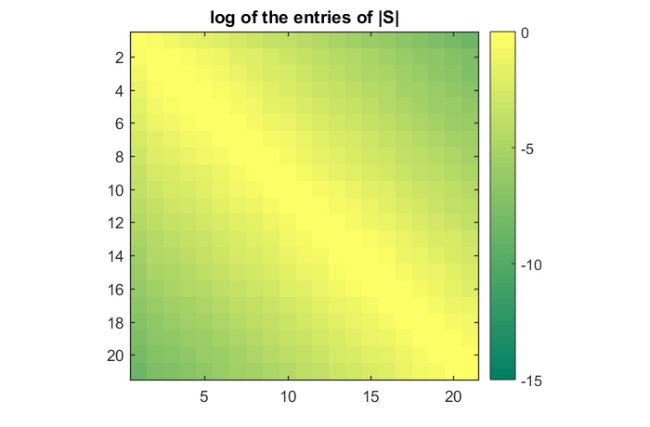The projection is not tridiagonal, but it is semiseparable! A semiseparable matrix is one for which any submatrix consisting of elements in the strictly lower (upper, due to symmetry in this case) part is of rank at most $1$.

disp([rank(S(3:m, 1:2),  1e-15), ...
rank(S(4:m, 1:3),  1e-15), ...
rank(S(7:12, 1:4), 1e-15)])

     1     1     1


Note that $S_{m+1}$ is nonsingular and its inverse is tridiagonal.

figure(3), colormap('summer')
imagesc(log10(abs(S\eye(m+1))))
colorbar, set(gca, 'CLim', [-15, 0]); axis square
title('log of the entries of |inv(S)|')## Semiseparable plus diagonal matrices

We now consider a more general rational Krylov space, having both finite and infinite poles. The ordering of the poles is irrelevant for the final space, but the structure of the projection may change depending on it.

Specifically, we look at a rational Krylov space with $m=12$ poles $\xi_j$ appearing in four groups. The first group consists of three poles at infinity, the second contains three finite poles, two infinite poles make the third group, and in the fourth there are four finite poles. The matrix $A$ is chosen as nonsymmetric.

A  = gallery('grcar', N);
xi = [inf, inf, inf, ...
-20, -10, 80,  ...
inf, inf,      ...
-20, 80, 80, -50];


The structure of $V_{m+1}^*AV_{m+1}^{\phantom{.}}$ is related to the pole groups. Define the diagonal matrix of poles $D_{m+1}$ by setting $d_1=0$, and $d_{j+1}=\xi_j$ if $\xi_j\neq\infty$ or $d_{j+1}=0$ if $\xi_j=\infty$, for $j=1, \ldots, m$. Then the matrix $S_{m+1} = V_{m+1}^*AV_{m+1}^{\phantom{.}}-D_{m+1}^{\phantom{.}}$ is semiseparable.

[V, K, H] = rat_krylov(A, b, xi);
S = V'*A*V;
D = diag([0 xi]); D(D == inf) = 0;
S = S - D;

figure(4), colormap('summer')
imagesc(log10(abs(S)))
colorbar, set(gca, 'CLim', [-15, 0]); axis square
title('log of the entries of |S|')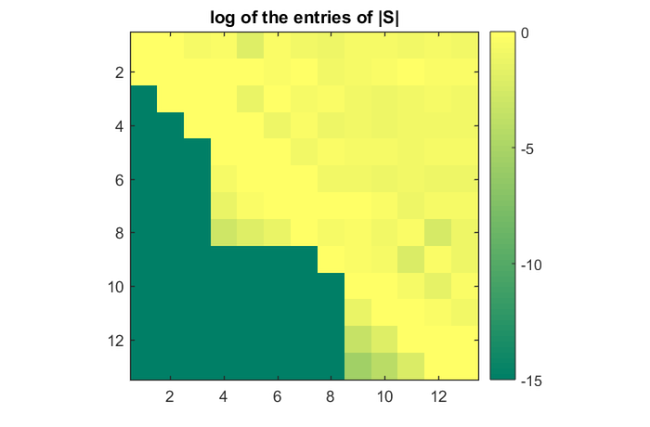For each of the four pole groups there is a corresponding submatrix of $S_{m+1}$. The submatrices lie on the diagonal of $S_{m+1}$ and share $2\times 2$ corner(s) with the neighbouring blocks. The two submatrices corresponding to the two groups with infinite poles are upper-Hessenberg, and the other two are inverse upper-Hessenberg.

l1 = line([0, 0, 5, 5, 0]+.5,   [0, 5, 5, 0, 0]+.5);
l2 = line([3, 3, 8, 8, 3]+.5,   [3, 8, 8, 3, 3]+.5);
l3 = line([6, 6, 10, 10, 6]+.5, [6, 10, 10, 6, 6]+.5);
l4 = line([8, 8, 13, 13, 8]+.5, [8, 13, 13, 8, 8]+.5);
set([l1,l2,l3,l4],'LineWidth',3)
set(l1,'Color','r'), set(l2,'Color','g')
set(l3,'Color','m'), set(l4,'Color','b')
set(gca, 'XTick', 1:13,'YTick',1:13)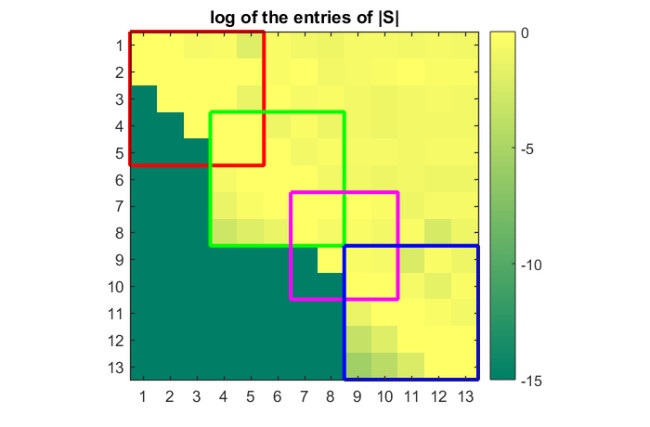In the following plot we show the inverses of the second and fourth block, to confirm that they are indeed upper Hessenberg matrices.

figure(5), colormap('summer')
subplot(121)
imgsci = @(X, ii) imagesc(log10(abs(X(ii, ii)\eye(length(ii)))));
imgsci(S, 4:8), set(gca,'CLim',[-15,0]); axis square
set(gca,'XTick',1:5,'XTickLabel',4:8,'YTick',1:5,'YTickLabel',4:8)
title('|inv(S(4:8, 4:8))|')

subplot(122)
imgsci(S, 9:13), set(gca,'CLim',[-15,0]); axis square
set(gca,'XTick',1:5,'XTickLabel',9:13,'YTick',1:5,'YTickLabel',9:13)
title('|inv(S(9:13, 9:13))|')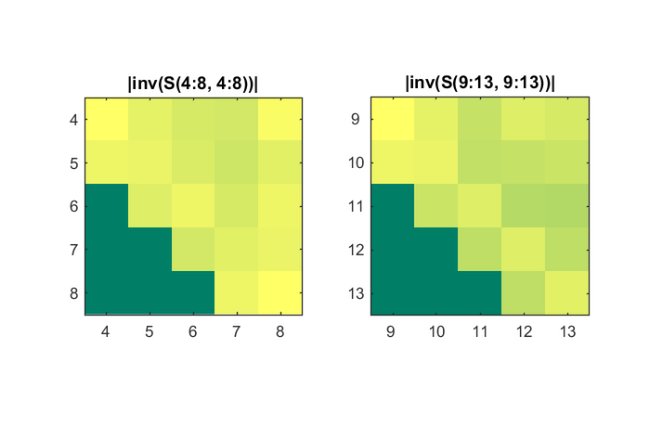We now relocate the poles of the above rational Krylov space, as described in , and visualize the semiseparable structure. We take $6$ groups of poles and can spot the corresponding overlapping upper-Hessenberg and inverse upper-Hessenberg blocks.

xi_new = [-20, -30,  ...
inf, inf, inf, ...
-30, -20,      ...
inf, inf,      ...
-30,           ...
inf, inf];

D_new = diag([0 xi_new]); D_new(D_new == inf) = 0;
[~, ~, Q] = move_poles_expl(K, H, xi_new);
S = Q*(S+D)*Q'-D_new;

figure(6), colormap('summer')
imagesc(log10(abs(S))), colorbar, set(gca, 'CLim', [-15, 0]);
axis square, title('log of the entries of |S|')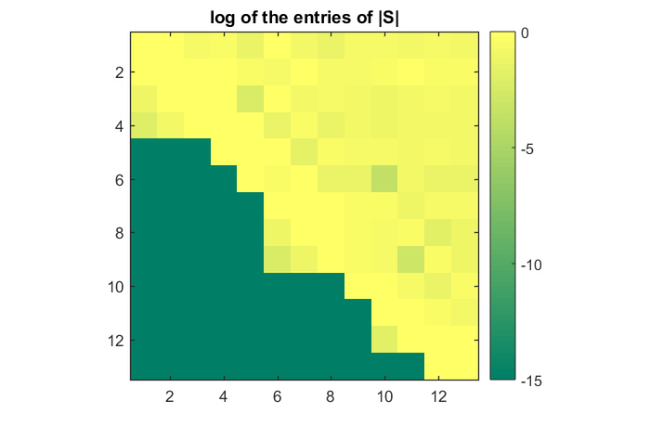The connection between rational Krylov spaces and semiseparable (plus diagonal) matrices has been used, for instance, for the development of short recurrences in rational quadrature rules, see e.g. [3, 6] and the references given therein. In [4, 5] the authors used it to approximate a rational Krylov space from a larger polynomial Krylov space. The examples shown here are for rather small matrices and low-dimensional rational Krylov spaces. For larger examples the semiseparable structure is often obscured by numerical roundoff and not reliably exploited, which is one of the reasons we prefer to work with the upper-Hessenberg representations for rational Krylov spaces, see e.g. [1, 7].

The following command is used to create a thumbnail.

figure(4), colorbar off, axis square, axis off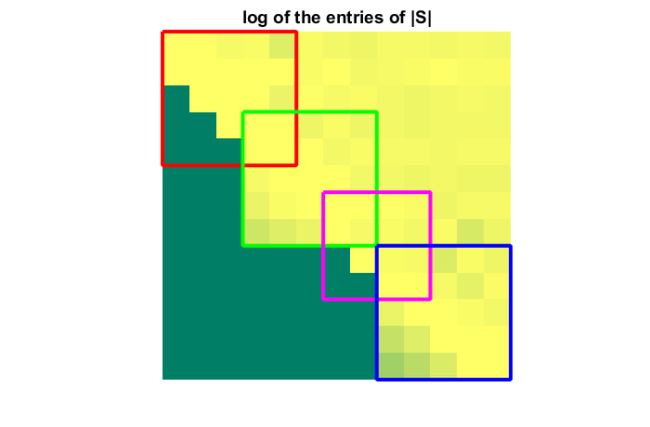## References

 M. Berljafa and S. Güttel. Generalized rational Krylov decompositions with an application to rational approximation, SIAM J. Matrix Anal. Appl., 36(2):894--916, 2015.

 D. Fasino. Rational Krylov matrices and QR steps on Hermitian diagonal-plus-semiseparable matrices, Numer. Linear Algebra Appl., 12(8):743--754, 2005.

 C. Jagels and L. Reichel. Recursion relations for the extended Krylov subspace method, Linear Algebra Appl., 434(7):1716--1732, 2011.

 T. Mach, M. Pranic, and R. Vandebril. Computing approximate extended Krylov subspaces without explicit inversion, Electron. Trans. Numer. Anal., 40:414--435, 2013.

 T. Mach, M. Pranic, and R. Vandebril. Computing approximate (block) rational Krylov subspaces without explicit inversion with extensions to symmetric matrices, Electron. Trans. Numer. Anal., 43:100--124, 2014.

 M. Pranic and L. Reichel. Rational Gauss quadrature, SIAM J. Numer. Anal., 52(2):832--851, 2014.

 A. Ruhe. Rational Krylov: A practical algorithm for large sparse nonsymmetric matrix pencils, SIAM J. Sci. Comput., 19(5):1535--1551, 1998.

 M. Van Barel, D. Fasino, L. Gemignani, and N. Mastronardi. Orthogonal rational functions and structured matrices, SIAM J. Matrix Anal. Appl., 26(3):810--829, 2005.